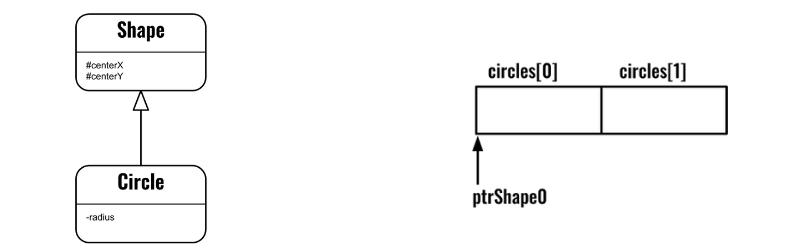# Pointer arithmetic on base class pointer

A class Circle inherits class Shape as follows:

``````class Shape {
public:
//.. methods ..
protected:
int centerX=0, centerY=0;
};

class Circle : public Shape {
public:
//.. methods ..
private:
};
``````

An array of Circles is defined and a base class pointer is pointed to the first element in the array:

``````Circle circles;
Shape* ptrShape0 = &circles;
``````

The illustration below depicts the relations between Shape and Circle, and also where the ptrShape0 is pointing in the array circles:Another base class (Shape) pointer, ptrShape1, is initialized by incrementing ptrShape0 as follows:

``````auto ptrShape1 = ptrShape0 + 1;
``````

Which one of the following expressions will return true?